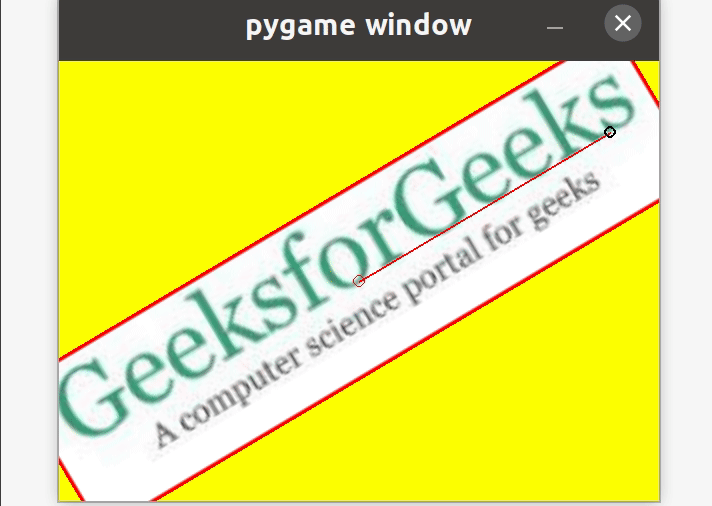How to use the mouse to scale and rotate an image in PyGame ?

• Last Updated : 28 Apr, 2021

In this article, we will discuss how to transform the image i.e (scaling and rotating images) using the mouse in Pygame.

Approach

Step 1: First, import the libraries Pygame and math.

import pygame
import math
from pygame.locals import *

Step 2: Now, take the colors as input that we want to use in the game.

color_1 = #RGB value of color 1
color_2 = #RGB value of color 2
color_n = #RGB value of color n

Step 3: Then, initialize the constructor

pygame.init()

Step 4: Set the dimensions of your GUI game.

w, h = #Width dimension, #Height dimension
screen = pygame.display.set_mode((w, h))

Step 5: Set the running value for running the game, the angle by which it can be moved.

running = True
angle = 0
scale = 1

Step 6: Next, take the image as input which we want to move with the mouse

img_logo = pygame.image.load('#Enter the image url')
img_logo.convert()

Step 7: Draw a border around an image.

rect_logo = img_logo.get_rect()
pygame.draw.rect(img_logo, color_1, rect_logo, 1)

Step 8: Locate the center of the GUI game and get the position of the mouse.

center = w//2, h//2
mouse = pygame.mouse.get_pos()

Step 9: Store the image in a new variable and construct a rectangle around the image.

img = img_logo
rect = img.get_rect()
rect.center = center

Step 10: Set the things which you want your app to do when in running state.

while running:
for event in pygame.event.get():
• Step 10.1: Once the app is in a running state, make it quit if the user wants to quit.
if event.type == QUIT:
running = False
• Step 10.2: In case, the user doesn’t want to quit, set at what angle the image should rotate.
if event.type == KEYDOWN:
if event.key == K_ra:
if event.mod & KMOD_SHIFT:

# angle at which it should move left
angle -=
else:

# angle at which it should move right
angle +=
• Step 10.3: Also, set at what ratio the image size should decrease or increase.
elif event.key == K_sa:
if event.mod & KMOD_SHIFT:
scale /= #scale at which the image size should decrease
else:
scale *= #scale at which the image size should increase
• Step 10.4: Set at what coordinates, angle, and scale the image will rotate or resize.
elif event.type == MOUSEMOTION:
• Step 10.4.1: Store the current position of the event in the new variable.
mouse = event.pos
• Step 10.4.2: Locate the Cartesian coordinates with the help of the mouse variable and center of the image for rotating the image..
x = mouse - center
y = mouse - center
• Step 10.4.3: Further, calculate the distance between the two points (0,0) and (x, y) with the help of formula √x2+y2
d = math.sqrt(x ** 2 + y ** 2)
• Step 10.4.4: Now, calculate the angle in degrees at which the image should rotate using the Python method math.atan2() which returns the arctangent of y/x, in radians.
angle = math.degrees(-math.atan2(y, x))
• Step 10.4.5: Calculate which scale the image size should decrease or increase using the function abs, which returns the magnitude of the number.
scale = abs(5 * d / w)
• Step 10.4.6: Calculate the updated position of the image in the running state using the rotozoom function which is a combined scale and rotation transform.
img = pygame.transform.rotozoom(img_logo, angle, scale)
• Step 10.4.7: Construct the rectangle around the updated image
rect = img.get_rect()
rect.center = center

Step 11: Next, you need to set the screen color and the image on the screen.

screen.fill(color_3)
screen.blit(img, rect)

Step  12: Later on, draw the rectangle, line, and circles which will help in moving the image.

pygame.draw.rect(screen, color_2, rect, #thickness of rectangle)
pygame.draw.line(screen, color_3, center, mouse, #Thickness of line)
pygame.draw.circle(screen, color_1, center, #radius of circle, #thickness of circumference)
pygame.draw.circle(screen, color_2, mouse, #radius of circle, #thickness of circumference)

Step 13: Furthermore, update the changes done in the GUI game.

pygame.display.update()

Step 14: Finally, quit the GUI game.

pygame.quit()

Below is the implementation.

Python

 # Python program to transform the # image with the mouse  #Import the libraries pygame and mathimport pygameimport mathfrom pygame.locals import *  # Take colors inputRED = (255, 0, 0)BLACK = (0, 0, 0)YELLOW = (255, 255, 0)  #Construct the GUI gamepygame.init()  #Set dimensions of game GUIw, h = 600, 440screen = pygame.display.set_mode((w, h))  # Set running, angle and scale valuesrunning = Trueangle = 0scale = 1  # Take image as inputimg_logo = pygame.image.load('gfg_image.jpg')img_logo.convert()  # Draw a rectangle around the imagerect_logo = img_logo.get_rect()pygame.draw.rect(img_logo, RED, rect_logo, 1)  # Set the center and mouse positioncenter = w//2, h//2mouse = pygame.mouse.get_pos()  #Store the image in a new variable#Construct the rectangle around imageimg = img_logorect = img.get_rect()rect.center = center  # Setting what happens when game is# in running statewhile running:    for event in pygame.event.get():          # Close if the user quits the game        if event.type == QUIT:            running = False          # Set at which angle the image will        # move left or right        if event.type == KEYDOWN:            if event.key == K_ra:                if event.mod & KMOD_SHIFT:                    angle -= 5                else:                    angle += 5              # Set at what ratio the image will            # decrease or increase            elif event.key == K_sa:                if event.mod & KMOD_SHIFT:                    scale /= 1.5                else:                    scale *= 1.5                          # Move the image with the specified coordinates,        # angle and scale                elif event.type == MOUSEMOTION:            mouse = event.pos            x = mouse - center            y = mouse - center            d = math.sqrt(x ** 2 + y ** 2)            angle = math.degrees(-math.atan2(y, x))            scale = abs(5 * d / w)            img = pygame.transform.rotozoom(img_logo, angle, scale)            rect = img.get_rect()            rect.center = center          # Set screen color and image on screen    screen.fill(YELLOW)    screen.blit(img, rect)      # Draw the rectangle, line and circle through    # which image can be transformed     pygame.draw.rect(screen, BLACK, rect, 3)    pygame.draw.line(screen, RED, center, mouse, 2)    pygame.draw.circle(screen, RED, center, 6, 1)    pygame.draw.circle(screen, BLACK, mouse, 6, 2)          # Update the GUI game    pygame.display.update()  # Quit the GUI gamepygame.quit()

Output:My Personal Notes arrow_drop_up